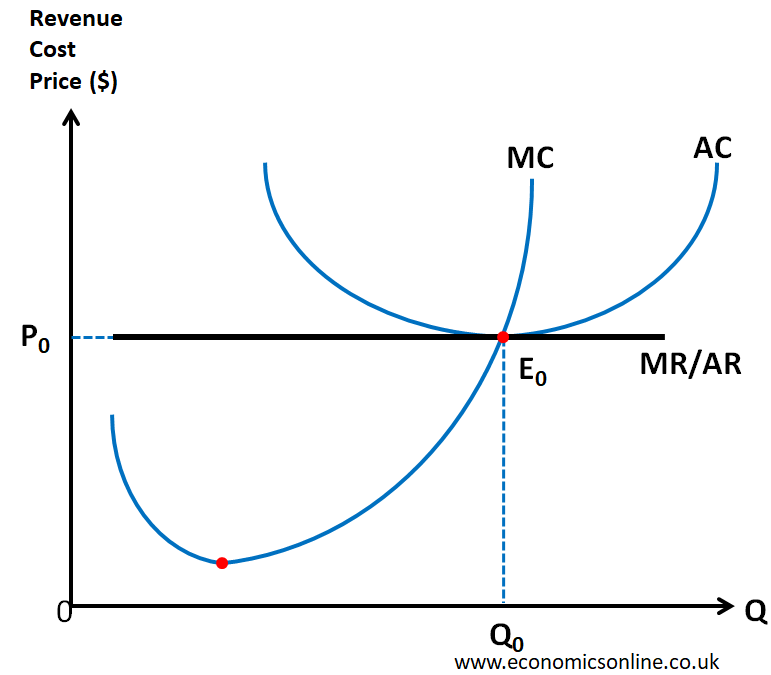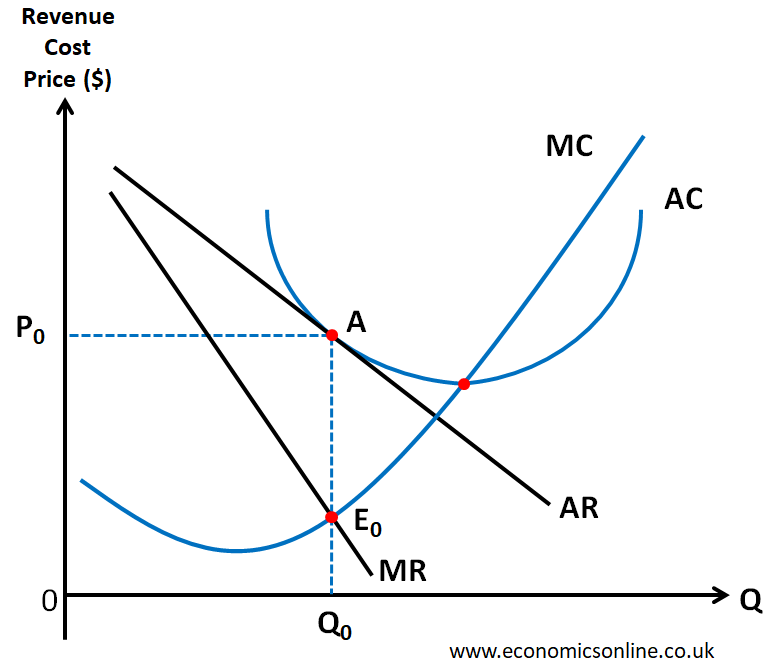# Allocative Efficiency

The resources of a country are said to be efficiently allocated if they are being used in the "best" possible way. This means that the maximum of the infinite wants of people are satisfied by using scarce resources. This will lead to maximum overall satisfaction in the economy. This is called the efficient allocation of resources. Efficient resource allocation is always desirable because it represents the best possible solution to the economic problem (limited resources and unlimited wants). One way to achieve this is through allocative efficiency.

Allocative efficiency refers to the optimal distribution of goods in an economy to meet the needs and wants of society. It is a measure of how well an economy is using its resources to produce the goods and services that people value the most. The goal of allocative efficiency is to ensure that resources are used in such a way that their marginal benefit to society is equal to their marginal cost.

## Definition of Allocative Efficiency

Allocative efficiency means producing the output level as desired by the people of the country.

This can be achieved when the firms and markets have Allocative efficiency.

## Allocative Efficiency for a Firm

Allocative efficiency is said to exist for a firm producing the output for which the price is equal to the marginal cost of production (P=MC)

Consider the following data for a firm. The price of the product produced by the firm is \$3.

For output of 3 units, the price and the marginal cost (MC) are equal to \$3. If this firm will produce and sell 3 units, the condition of allocative efficiency (P = MC) is satisfied, and the firm is said to have allocative efficiency.

This same is shown by the following diagram when Allocative efficiency exists at point A which is the point of intersection of price and marginal cost curve.

## Allocative Efficiency for a Market

Markets have Allocative efficiency when the resources are used in such a way that the marginal social cost (MSC) is equal to the marginal social benefit (MSB). Hence, if MSC=MSB, then society’s welfare is maximized and allocative efficiency is said to exist.

Consider the following diagram:

For output Qs (the socially desirable output), MSC = MSB at point Es. If a market will produce this output Qs, allocative efficiency will exist in that market.

At output Q1, MSB > MSC, and there is under-allocation of resources. So output should be increased to achieve allocative efficiency.

At output Q2, MSB < MSC, and there is over- allocation of resources. So output should be decreased to achieve allocative efficiency.

## Allocative Efficiency in different Types of Markets

### Perfect Competition

The following diagram illustrates the long run equilibrium (profit maximization) of a firm operating in perfect competition at point E0 (point of equilibrium) where marginal revenue is equal to marginal cost (MR=MC) for output Q0.Graph illustrating allocative efficiency for a firm operating in perfect competition.

We can see that, at output Q0, P=MC at point E0 (point of allocational efficiency). Hence the firm has allocative efficiency in perfect competition.

### Monopolistic Competition

The following diagram illustrates the long run equilibrium of a firm operating in monopolistic competition at point E0 where marginal revenue is equal to marginal cost (MR=MC) for output Q0.Graph illustrating allocative efficiency for a firm operating in monopolistic competition.

We can see that, at output Q0, P = Q0A and MC = Q0E0

Since P ≠ MC, So the firms operating in monopolistic competition are not allocatively efficient.

### Monopoly

The following diagram illustrates the long run equilibrium of a firm operating in monopoly at point E0 where marginal revenue is equal to marginal cost (MR=MC) for output Q0.

We can see that, at output Q0, P = Q0A and MC = Q0E0

Since P ≠ MC, So the firms operating in monopoly are not allocatively efficient.

The same is true for the collusive and non-collusive models of oligopoly in which firms do not have allocative efficiency.

Hence, allocative efficiency exists only in case of perfect competition.

## Importance of Allocative Efficiency

Allocative efficiency is an important concept because it ensures that resources are used in the most efficient way possible. This leads to the satisfaction of the highest number of wants of people of the country.

## Other Types of Economic Efficiency

There are some other kinds of efficiency, which are briefly explained below.

### Productive Efficiency

For a firm or producer, productive efficiency means producing output at the lowest point of the lowest AC curve (Minimum AC).

For a country, productive efficiency means producing output at any point on its production possibility frontier (PPF). It means all firms in a country are productively efficient.

### Pareto Efficiency (Pareto Optimality)

A situation is called Pareto efficient if it is impossible to make someone better off without making someone else worse off.

## Is Allocative Efficiency Achievable?

Achieving allocative efficiency is not always easy. In real-world economies, there are often market failures that prevent the efficient allocation of resources. For example, market power can lead to monopolies and oligopolies, which can result in higher prices for consumers and lower levels of output. Similarly, externalities can result in under-allocation or over-allocation of resources leading to market failures.

## The Role of Government in achieving Allocative Efficiency

Governments can play a role in promoting allocative efficiency by addressing market failures. For example, antitrust policies can be used to prevent monopolies and oligopolies, while regulations can be used to address negative externalities. Additionally, government investment in education and infrastructure can help to increase the productive capacity of an economy, promoting allocative efficiency in the long run.

## Conclusion

In conclusion, allocative efficiency is a crucial concept in economics that refers to the optimal distribution of resources in an economy to meet the needs and wants of society. The goal of allocative efficiency is to ensure that resources are used in such a way that their marginal benefit to society is equal to their marginal cost. Market-oriented economies strive to achieve allocative efficiency through the functioning of market prices, but real-world economies often face market failures that prevent the efficient allocation of resources. Governments can play a role in promoting allocative efficiency by addressing market failures and investing in education and infrastructure.